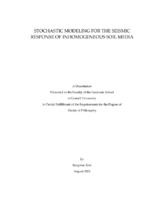## Stochastic Modeling for the Seismic Response of Inhomogeneous Soil Media##### Abstract
A method is developed for generating synthetic seismic ground motion records. The records are solutions of stochastic wave equations with deterministic inputs, which correspond to specified fault ruptures, and random coefficients, which capture spatial random fluctuations of soil properties. Matrix-valued homogeneous non-Gaussian random fields are constructed to characterize soil properties. The numerical solution of stochastic wave equations involves two steps. First, the unbounded domain of definition is replaced with a bounded subdomain with appropriate boundary conditions to limit wave reflection. Second, the resulting spatial domain is discretized. The solution of the wave equation is approximated by a state vector whose components are the values of this solution at the nodes of the spatial discretization. The state vector satisfies a linear ordinary differential equation with random coefficients, which is solved by random vibration method and Monte Carlo simulation. Statistical results of seismic ground motions are presented for various types of soil layers.
184 pages
2021-08
##### Keywords
Ground motion; Inhomogeneous soil media; Monte Carlo simulation; Random soil model; Stochastic wave equation
##### Committee Chair
Grigoriu, Mircea Dan
##### Committee Member
Earls, Christopher J.
Frazier, Peter
##### Degree Discipline
Civil and Environmental Engineering
##### Degree Name
Ph. D., Civil and Environmental Engineering
##### Degree Level
Doctor of Philosophy